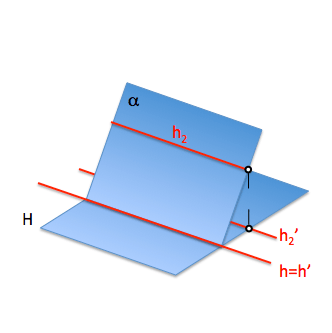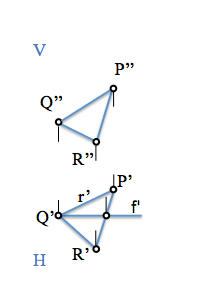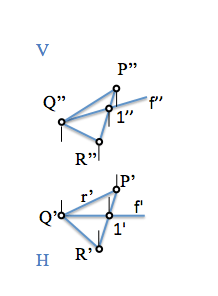# Diédrico System: Straight lines in a plane parallel to the projection

We will see that these lines are useful in the operation that we are going to be developed in the study of the dihedral system, Since we make it easy, inter, the determination of directions for obtaining new views, the direct determination of perpendicular, the obtaining of angles etc..

These straight lines fall under the so-called category “notable lines” plane and may belong to three different categories:

• Straight lines parallel to the planes of projection
• Straight lines perpendicular to the planes of projection
• Straight lines that form the maximum angular condition with respect to the planes of projection.

We will start studying those straight lines that are parallel to the planes of projection.

• Horizontal plane (parallel to the plane Horizontal H)
• Front plane (parallel to the plane of Vertical V)
• Straight profile (parallel to the plane of profile P)

In all cases these lines have in common that the distance of all points to one of the planes of projection, which are parallel, It will be the same and in this projection will be in true extent.1. If the straight line is horizontal, its projection h’ will be seen in true extent. This line will be parallel to the intersection of the plane with the horizontal plane H.
2. If the straight line is front, its projection f” will be seen in true extent. This line will be parallel to the intersection of the plane with the vertical plane V...
3. If the line is of profile, its projection p”’ will be seen in true extent. This line will be parallel to the intersection of the plane with the plane's profile P

We see that we can study for one of the specific positions, for example parallel to the horizontal, and generalize the parallelism with regard to other levels simply by rotating the projections.

### Horizontal plane

The relative dimension of all points (z) remains constant, by which we can determine its vertical projection knowing that its direction is perpendicular to the reference lines between the vertical plane (V..) and the horizontal plane (H)To obtain the projection simply missing will use the concepts of belonging that we have seen for obtain points in the plane. To belong to the straight “h” to the plane, will belong to your points (“Q” and “1”)We see that in the new projection on the horizontal plane, the straight line is seen in true extent.

### Front of plane

The relative distance of all points (and) remains constant, by which we can determine its horizontal projection knowing that its direction is perpendicular to the reference lines between the vertical plane (V..) and the horizontal plane (H)To obtain the projection simply missing will use the concepts of belonging that we have seen for obtain points in the plane. To belong to the straight “f” to the plane, will belong to your points (“Q” and “1”)We see that in the new projection on the horizontal plane, the straight line is seen in true extent.

### Straight profile

Only we would determine the straight lines that are parallel to the profile, for what we need to determine this third projection. Is left to the reader its determination so you generalize these elementary concepts studied. Do you get it?

Sistemas_de_representacion